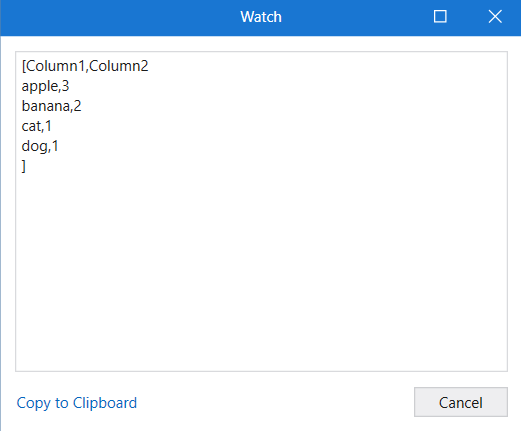# From a list of strings to get the count of similar items

I have a list of values, and I want to count of the similar value in the list stored In data table.

Ex:
Input:
ListA={apple, apple, banana, apple, cat, banana, dog}

Output:

Col1. col2
apple. 3
banana. 2
cat. 1
dog. 1

I need a linq query that achieves the above output?

Possible??

The below code you can use it to get the name and count of the element in the list. It will return as a dictionary type

``````ListA.GroupBy(Function(x) x).ToDictionary(Function(x) x.Key, Function(x) x.Count())
``````

Hi,

Can you try the following sample?

First, define dt which has 2 columns using Build DataTable activity.

Then

``````dt = yourList.GroupBy(Function(s) s).Select(Function(g) dt.LoadDataRow({g.Key,g.Count},False)).CopyToDataTable()
``````

Sequence.xaml (8.0 KB)

Regards,

Checkout this expression

``````(From d In sample
Group d By k=d.ToString.Trim Into grp=Group
Let f= grp(0)
``````

Regards
Sudharsan

1 Like

Hi @Ray_Sha1 ,

Can you try this,

(From x In list.AsEnumerable().GroupBy(Function(x) x).ToList
Let y = list.AsEnumerable().Where(Function(a) a.ToString=x(0).ToString).Count